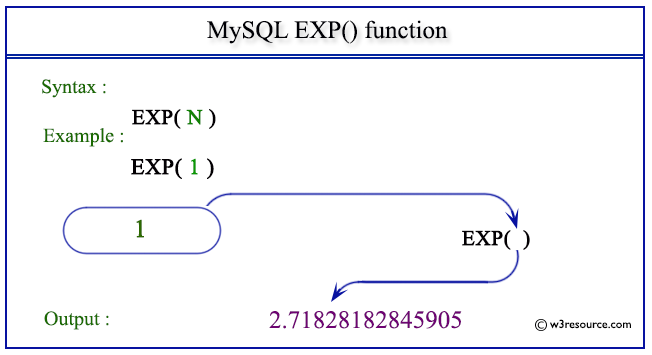# MySQL EXP() function

## EXP() function

MySQL EXP() returns the value of the base of natural logarithm number e, raised to the power of a number specified as argument.

Syntax:

```EXP(N);
```

Argument

Name Description
N A number.

Syntax Diagram:MySQL Version: 5.6

Pictorial presentation of MySQL EXP() functionExample of MySQL EXP() function

Code:

``````SELECT EXP(1);
```
```

Explanation

The above MySQL statement will return the value of the natural logarithm base number e raised to the power of the given number.

Sample Output:

```mysql> SELECT EXP(1);
+------------------+
| EXP(1)           |
+------------------+
| 2.71828182845905 |
+------------------+
1 row in set (0.03 sec)
```

Example: EXP() function with negative value

Code:

``````SELECT EXP(-1);
```
```

Explanation

The above MySQL statement will return the value of the natural logarithm base number e raised to the power of the given number.

Sample Output:

```mysql> SELECT EXP(-1);
+-------------------+
| EXP(-1)           |
+-------------------+
| 0.367879441171442 |
+-------------------+
1 row in set (0.00 sec)
```

All Mathematical FunctionsPrevious: DIVISION
Next: FLOOR()

﻿